# Requests & Ideas - page 29163387

Halvard

You are right, my mmistake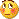Either change line 65 from
IndicatorBuffers(2);
to
IndicatorBuffers(3);
Or use the one attached here (checked it and that is the only change that needs to be doe - now zero line crosses are working too as they should)

regards

halvardu:

It dont seems like the zerro alert it working? can you please check it?

Halvard
Files:131

Halvard

You are right, my mmistakeEither change line 65 from
IndicatorBuffers(2);
to
IndicatorBuffers(3);
Or use the one attached here (checked it and that is the only change that needs to be doe - now zero line crosses are working too as they should)

Thank you Mladen! Its working perfect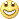Regard

Halvard339

...............110

PS : Zipfrog, will do it. Right now I have some things to do that I can not postpone but will finish it soon and then will make what you requested

Regards,

Zipfrog118

could u possibly make the dinapoli macd predictor and oscillator predictor? here is the documentation:

thanks

odysseus163387

odysseus

This is a first step towards DiNapolli levels (as a part of the whole package) Been looking for reliable formulas about the whole package (formulas, not descriptions how do they work on charts) and so far found just one book describing in more detail the whole thing - for example DiNapolli MACD is a MACD with smoothing factors of .213, .108, and .199)

_________________________

Anyway decided to start with the most difficult one today (but will probably make the easier ones along)

It turns out (at least from the literature I have found so far and which can be considered a serious one) that D-levels depend entirely on Elliot wave. Now, Elliot wave is a "terra incognita" (even for ZUP indicator as far as I see, since nen (the author of "zup" indicator) asked a question from metatrader 5 coders how do they identify (the algorithm) Elliot wave 3 and Elliot wave 5, and the answer was that it is just an object that is drawn by hand - user is clicking at peaks he finds suitable)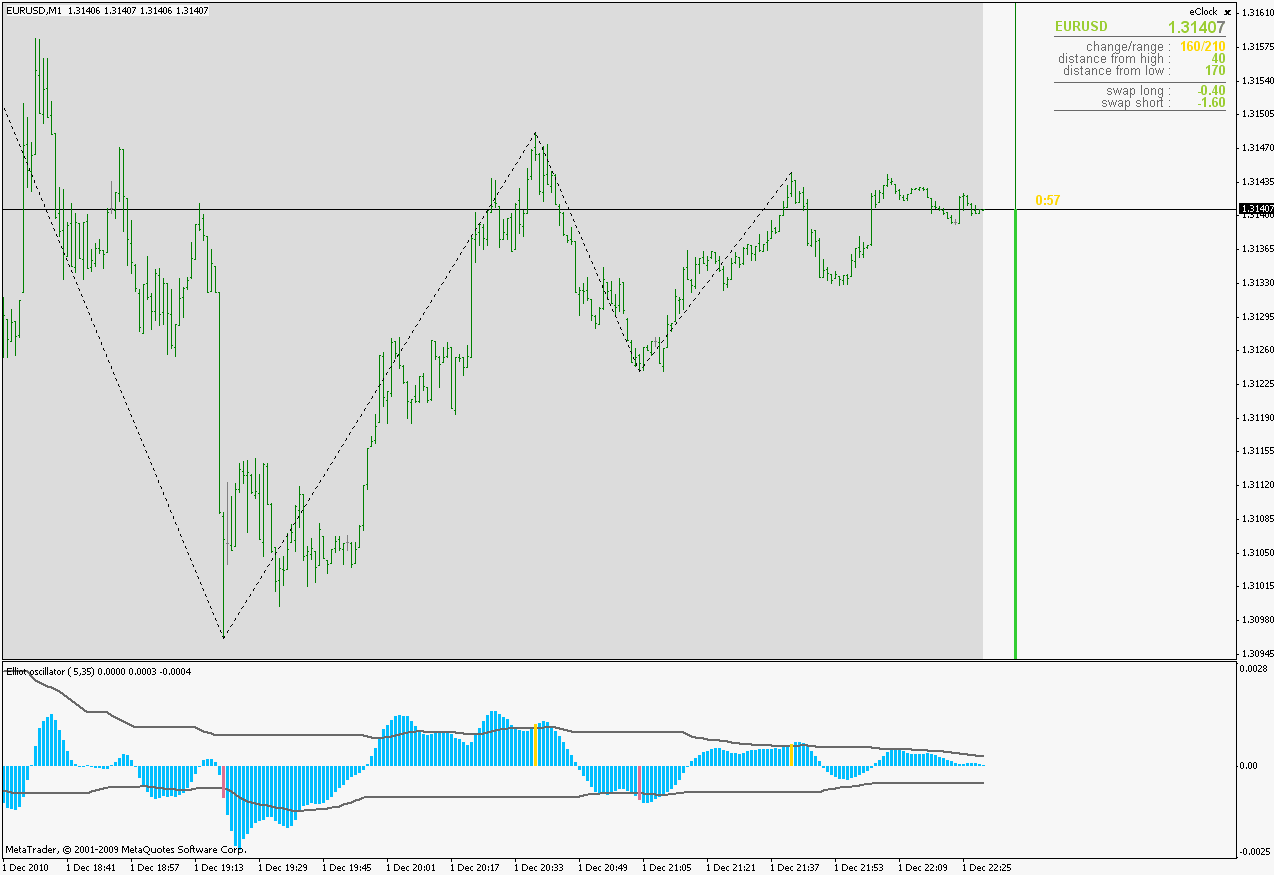This is a try to find a reliable way of identifying waves. The idea is simple : to use Elliot oscillator, but the advanced get variation, for peaks identifying. So this is the first step towards it. You can choose how many peaks you want it to find, The counting part (which is the essence of Elliot waves) has not been done yet - that is the next step.

When this becomes a reliable Eliot wave indicator then the D-levels are rather simple (the simplest D-levels are 3 Fibonacci levels calculated from last Elliot wave) It seems that the first step is the most difficult one regarding D-levels

_________________________

PS: as every peak finding code, this will recalculate (since I do not like the use of word "repaint" for a code that calculate values correctly, "repainting" is in my opinion a coding error) so please bare in mind that

regards

odysseus11:

could u possibly make the dinapoli macd predictor and oscillator predictor? here is the documentation:

thanks

odysseus
Files:118

wow thanks mladen this is a great start - look forward to more !

odysseus339

odysseus

This is a first step towards DiNapolli levels (as a part of the whole package) Been looking for reliable formulas about the whole package (formulas, not descriptions how do they work on charts) and so far found just one book describing in more detail the whole thing - for example DiNapolli MACD is a MACD with smoothing factors of .213, .108, and .199)

_________________________

Anyway decided to start with the most difficult one today (but will probably make the easier ones along)

It turns out (at least from the literature I have found so far and which can be considered a serious one) that D-levels depend entirely on Elliot wave. Now, Elliot wave is a "terra incognita" (even for ZUP indicator as far as I see, since nen (the author of "zup" indicator) asked a question from metatrader 5 coders how do they identify (the algorithm) Elliot wave 3 and Elliot wave 5, and the answer was that it is just an object that is drawn by hand - user is clicking at peaks he finds suitable)This is a try to find a reliable way of identifying waves. The idea is simple : to use Elliot oscillator, but the advanced get variation, for peaks identifying. So this is the first step towards it. You can choose how many peaks you want it to find, The counting part (which is the essence of Elliot waves) has not been done yet - that is the next step.

When this becomes a reliable Eliot wave indicator then the D-levels are rather simple (the simplest D-levels are 3 Fibonacci levels calculated from last Elliot wave) It seems that the first step is the most difficult one regarding D-levels

_________________________

PS: as every peak finding code, this will recalculate (since I do not like the use of word "repaint" for a code that calculate values correctly, "repainting" is in my opinion a coding error) so please bare in mind that

regards

Great work Mladen ,top mark ,thank you.

What are those black lines ,are they standart deviation?Can you show these lines in the code?

Also can you make these levels adjustable?Thank you.163387

In the man time :

Dinapoli MACD. DiNapoli is using smoothing factors of 0.213, 0.108, and 0.199, which when translated to periods we are used to becomes 8.3896, 17.5185 and 9.0503. So here is this one that can work with fractional periods and is set by default to calculate DiNapoli MACD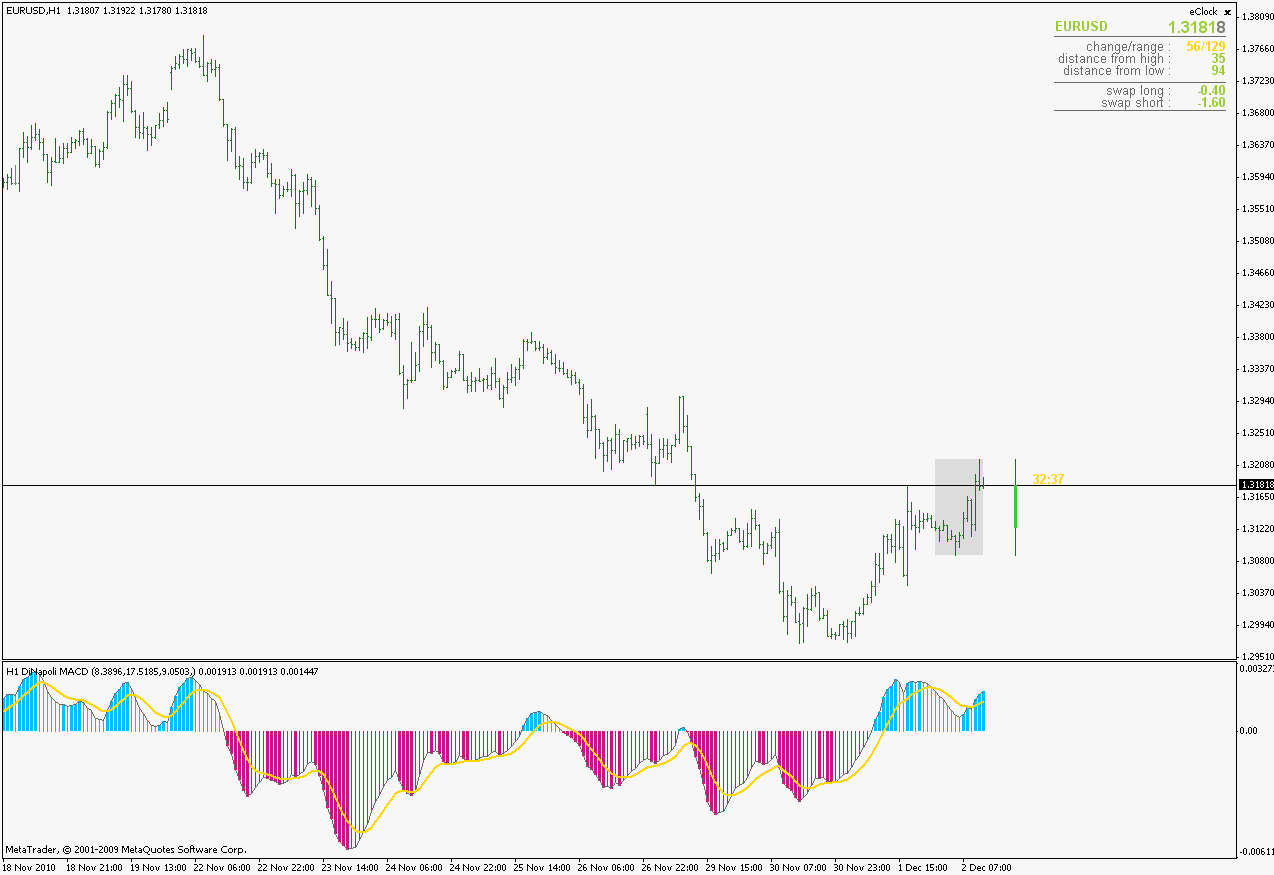biddick :

I am actually glad you asked that question. The black lines are a proof that simple ideas can be very effective (the original idea is not mine, as far as I know it is the idea from advanced get people but I am not 100% sure about it so don't take my word for it)

It is actually an EMA, but an EMA that is calculated for values that are "on the right side" of the zero line. So if the value is "on the right side" EMA is calculated as usually, but if the side is wrong, the value is simple inherited. It would be as if you removed every "empty" bar for values over 0 and calculate EMA on that value and doing the same for values less than zero. It is changeable : EMA period is calculated from shortPeriod and longPeriod - if you change either of it EMA will be calculated differently (but bare in mind that the original Elliot oscillator is calculated with those periods)

regards

Files:163387

Continuation of the Elliot oscillator - waves indicator

______________________

What has been done :
No limit in waves (now it draws waves for the whole history) Changed a logic a bit. It affects the way how extremes are found in some cases. The basic logic is the following : when (if) the oscillator value breaks the EMA value up or down then a new wave is started if the direction of the cross is oposite to the current wave direction (crossing zero line does not start a wave nor it ends the current wave). The beginning of the wave is at the first value belonging to that wave that crosses the zero value in the direction of the wave. Here is an example : the highest bar is not marked as extreme simply because it was still not confirmed by the oscillator value that it belongs to up wave.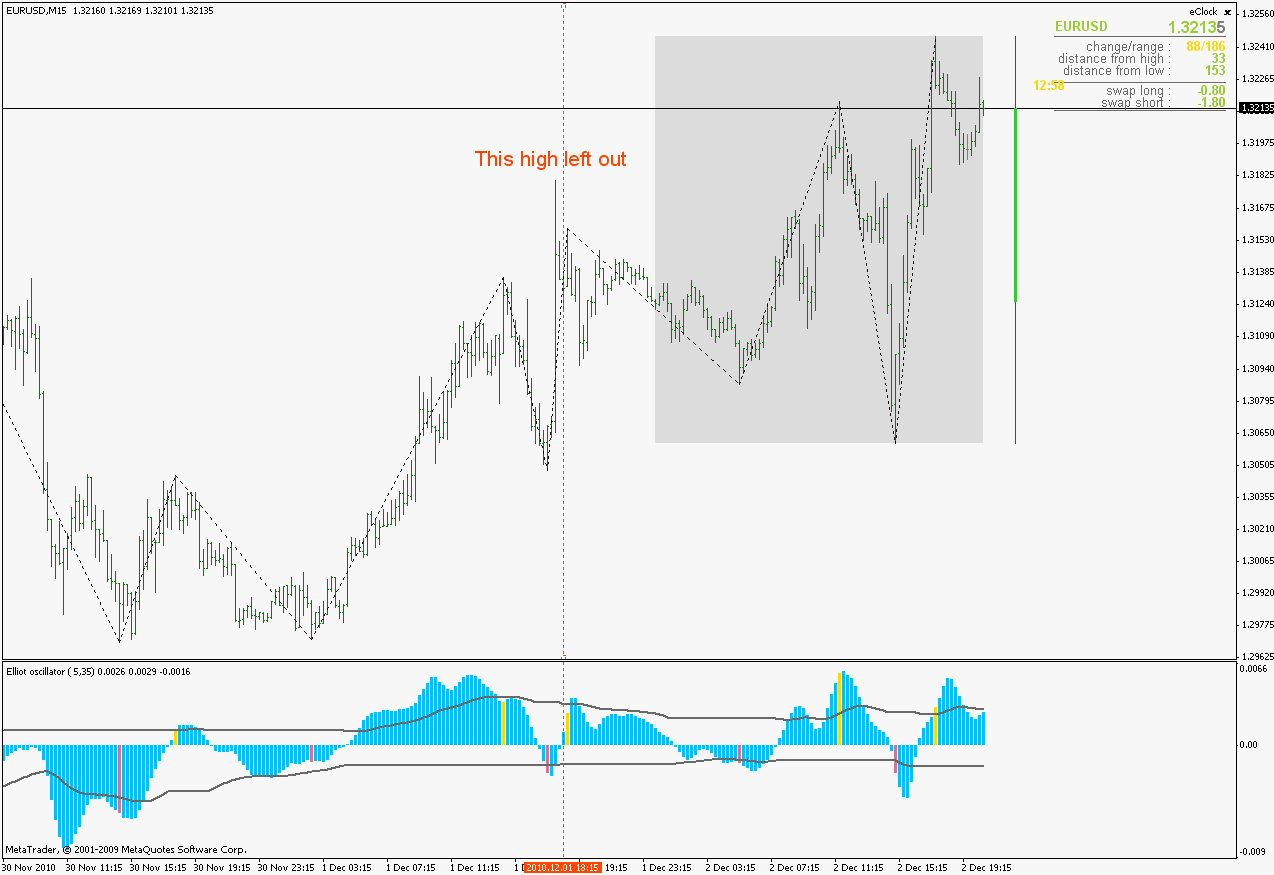Optimized the work (it is faster now - it calculates effectively just the last leg of the wave (it is necessary because of the changes that can happen to the last leg))
______________________

Also, as a note : only the last leg of the wave can change if new high or new low is reached. Once it is "opened" it can not (and I repeat) it can not disappear (like in some zigzags or some peak finding indicators), it can only go higher or lower if the price moves in the right direction. Its starting point is always going to stay fixed. So in combination with the oscillator itself I think it can be a useful tool

Files: